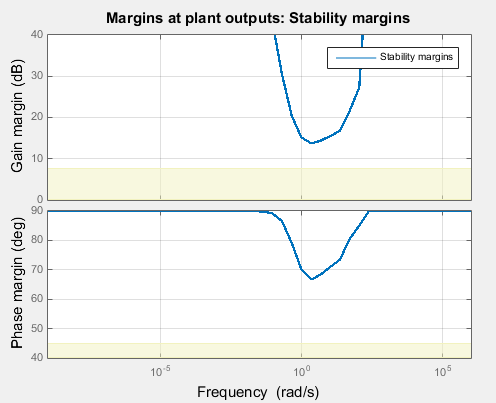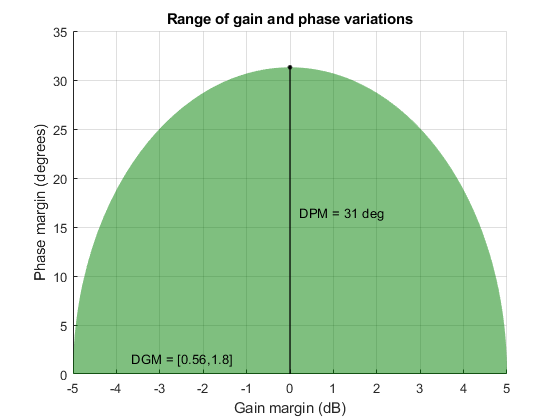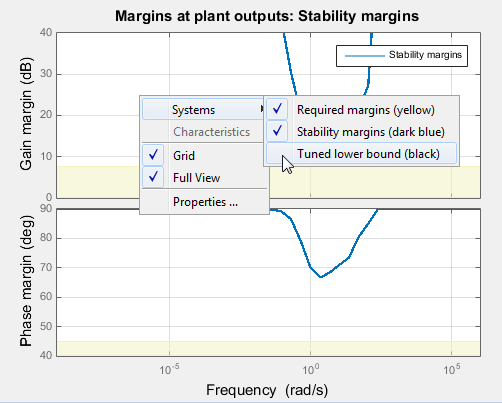## Stability Margins in Control System Tuning

When you visualize stability-margin goals in Control System Tuner or with `viewGoal`, the margins are displayed as a function of frequency. For instance, the following plot shows a typical result of tuning a control system with `systune` or Control System Tuner when you use a tuning goal that constrains stability margins... You obtain this plot in one of the following ways:

• Tuning in Control System Tuner using a Margins Goal or Quick Loop Tuning.

• Tuning at the command line using `systune` with `TuningGoal.Margins`. If `S` is the control system model or `slTuner` interface, and `Req` is a `TuningGoal.Margins` goal, obtain the stability-margin plot by entering:

`viewGoal(Req,S)`

The plot shows that how much gain or phase variation a system can tolerate without going unstable can depend on the frequency of the perturbation.

### Gain and Phase Margins

For SISO systems, the gain and phase margins at a frequency ω indicate how much the gain or phase of the open-loop response L() can change without loss of stability. For example, a gain margin of 5dB at 2 rad/s indicates that closed-loop stability is maintained when the loop gain increases or decreases by as much as 5dB at this frequency. Gain and phase margins typically vary across frequencies.

For MIMO systems, gain and phase margins are interpreted as follows:

• Gain margin: Stability is preserved when the gain increases or decreases by up to the gain margin value in each channel of the feedback loop.

• Phase margin: Stability is preserved when the phase increases or decreases by up to the phase margin value in each channel of the feedback loop.

In MIMO systems, the gain or phase can change in all channels at once, and by a different amount in each channel. The Margins Goal and `TuningGoal.Margins` rely on the notion of disk margin for MIMO systems. (See Stability Analysis Using Disk Margins (Robust Control Toolbox).) Like SISO stability margins, gain and phase margins in MIMO systems typically vary across frequency.

### Combined Gain and Phase Variations

To assess robustness to changes in both gain and phase, use the following chart.For example, if the gain margin plot in Control System Tuner indicates a 10 dB margin at a particular frequency, then trace the contour starting at `(Gain,Phase) = (10,0)` to see how a given amount of phase variation reduces the allowable gain variation at that frequency. For example, if the phase can vary by 30 degrees then the gain can only vary by about 8.4 dB (red mark).

### Interpreting the Gain and Phase Margin Plot

The stability-margin plot for Margins Goal or `TuningGoal.Margins` shows in shaded yellow the region where the target margins are not met. The plot displays the current gain and phase margins (computed using the current values of the tunable parameters in the control system) as a blue trace.

These gain and phase margin curves are obtained using an exact calculation involving μ-analysis. For computational efficiency, however, the tuning algorithm uses an approximate calculation that can yield smaller margins in parts of the frequency range. To see the lower bound used by the tuner, right-click on the plot, and select Systems > Tuned Lower Bound.If there is a significant gap between the true margins and the tuner approximation, try increasing the D-scaling order. The default order is zero (static scaling). For tuning in Control System Tuner, set the D-scaling order in the Margins Goal dialog box. For command-line tuning, set this value using the `ScalingOrder` property of `TuningGoal.Margins`.

### Algorithm

The gain and phase margin values are both derived from the disk margin. The disk margin measures the radius of a circular exclusion region centered near the critical point. (See Stability Analysis Using Disk Margins (Robust Control Toolbox).) This radius is a decreasing function of the scaled norm:

`$\underset{D\text{\hspace{0.17em}}\text{diagonal}}{\mathrm{min}}{‖{D}^{-1}\left(I-L\left(j\omega \right)\right){\left(I+L\left(j\omega \right)\right)}^{-1}D‖}_{2}.$`

Unlike the traditional gain and phase margins, the disk margins and associated gain and phase margins guarantee that the open-loop response L() stays at a safe distance from the critical point at all frequencies.